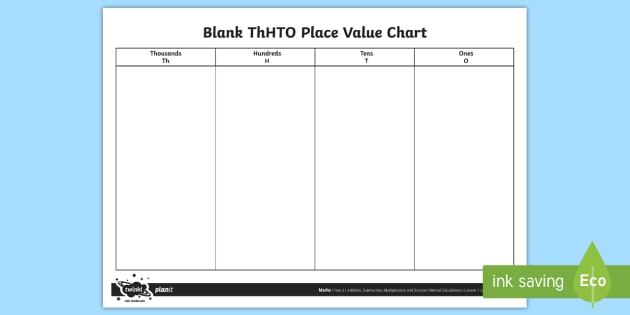# Multiplying in Maths

Tuesday 24 January 2023

By the end of Year 4, children are expected to know all multiplication tables
to 12 x 12 and recall the corresponding division facts. Using knowledge of
multiplication calculations, children will begin to use formal written methods to record their work, including the use of arrays. At home, you can support your child by practising reciting multiplication tables, ensuring your child can recall them and the corresponding division facts.

Today, some children used place value counters to help to multiply a two digit number by a one digit number.

34 x 4 =

4 lots of 34 =

Once the counters were in the correct place value rows, we looked to see whether we could exchange 10 ones for 1 ten and 10 tens for 1 hundred. Finally, we added up the total and wrote the answer. Can your child explain this to you?Recently, the class have been exploring how to  multiply by 10, 100 and 1,000. When multiplying by 10, children need to be able to visualise making a number 10 times the size and understand that “10 times the size” is the same as “multiply by 10”.

Key questions
• What do you notice when multiplying by 10?
• What is a placeholder? When do you use placeholders?
• What happens to the digits in a number when you multiply
by 10?
• What is multiplied by 10?
• What is 10 lots of ?

Help, to multiply by 10, at home by using this example changing the numbers each time.
7 × 10 = 70
10 × 7 = 70
70 is 10 times the size of 7

Is the statement always true, sometimes true or never true?
If you write a whole number in a place value chart and multiply it
by 10, all the digits move one column to the left.
Discuss this with your child. Can they recall the learning done in class?